Annu

Chicalim, Mormugao, India - 403711# Annu

## Maths Tutor

Chicalim, Mormugao, India - 403711.

3.8

UrbanPro Rating

Details verified of Annu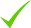IdentityEducation

Know how UrbanPro verifies Tutor details

Identity is verified based on matching the details uploaded by the Tutor with government databases.

## Overview

1 YEAR of teaching experience in Jaipur as a maths teacher and one year of experience in mumbai

English

Hindi

## Education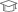Banasthali University 2015

Master of Science (M.Sc.)

## Address

Chicalim, Mormugao, India - 403711

## Verified Info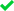Phone VerifiedEmail Verified

## Demo Class

No

Report this Profile

Is this listing inaccurate or duplicate? Any other problem?

Please tell us about the problem and we will fix it.

Please describe the problem that you see in this page.

Type the letters as shown below *

Please enter the letters as show below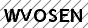## Class 6 Tuition Overview

Class 6 Tuition

Class LocationStudent's HomeTutor's Home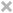Online (video chat via skype, google hangout etc)

Years of Experience in Class 6 Tuition

2

Board

CBSE

CBSE Subjects taught

Mathematics, Science

Taught in School or College

Yes

## Reviews

this is test message this is test message this is test message this is test message this is test message this is test message this is test message

No Reviews yet! Be the first one to Review

## Answers by Annu (3)

Answered on 27/12/2017

Radius and slant height of cone are given ,now first we will find height of cone with the help of pythagores theorem. P2+b2=h2 P2+(4)2=(5)2 P2+16=25 P2=25-16 P2=9 P=sqr root of 9 P=3 Volume of cone =1/3*pi*r2*h Volume =1/3*22/7*16*3 On solving this Volume of cone =16*pi
Answers 6 Comments
Dislike Bookmark

Answered on 21/12/2017

It will b 0 Since sin45=1/sqr root of 2 And cos45=1/sqr root of 2 Having the same value and if we perform operation Sin45-cos45 it will result into 0.
Answers 6 Comments
Dislike Bookmark

Answered on 21/12/2017

Let p(x)= x2+5x+6 Now putting p (x)=0 X2+5x+6=0 X2+3x+2x+6=0 X (x+3)+2 (x+3)=0 (X+3)(x+2)=0 (X+3)=0,(x+2)=0 X=-3,x=-2 Therefor -3 and -2 are zeros of given polynomial p (x)and names alpha and beta Now we have to verify the cofficients of given polynomial Comapre p (x)=ax2+bx+c ;a=1,b=5,c=6 Now alpha... ...more
Let p(x)= x2+5x+6 Now putting p (x)=0 X2+5x+6=0 X2+3x+2x+6=0 X (x+3)+2 (x+3)=0 (X+3)(x+2)=0 (X+3)=0,(x+2)=0 X=-3,x=-2 Therefor -3 and -2 are zeros of given polynomial p (x)and names alpha and beta Now we have to verify the cofficients of given polynomial Comapre p (x)=ax2+bx+c ;a=1,b=5,c=6 Now alpha +beta= -b/a Alpha*beta=c/a For the verification of cofficients take both the scenario L.H.S alpha+beta and R.H.S -b/a -3 + - 2 -5/1 -5 -5 Hence L.H.S=R.H.S Now Again check for L.H.S alpha*beta R.H.S c/a -3 * -2 6/1 6 6 Hence L.H.S = R.H.S So the relationship between zeros and cofficients of given polynomial is verified.
Answers 5 Comments
Dislike Bookmark

AnnuDirections

x
Class 6 Tuition 3.8

Class LocationStudent's HomeTutor's HomeOnline (video chat via skype, google hangout etc)

Years of Experience in Class 6 Tuition

2

Board

CBSE

CBSE Subjects taught

Mathematics, Science

Taught in School or College

Yes

Class 7 Tuition 3.8

Class LocationStudent's HomeTutor's HomeOnline (video chat via skype, google hangout etc)

Years of Experience in Class 7 Tuition

2

Board

CBSE

CBSE Subjects taught

Mathematics, Science

Taught in School or College

Yes

Class 8 Tuition 3.8

Class LocationStudent's HomeTutor's HomeOnline (video chat via skype, google hangout etc)

Years of Experience in Class 8 Tuition

2

Board

CBSE

CBSE Subjects taught

Mathematics, Science

Taught in School or College

Yes

Class 9 Tuition 3.8

Class LocationStudent's HomeTutor's HomeOnline (video chat via skype, google hangout etc)

Years of Experience in Class 9 Tuition

2

Board

CBSE

CBSE Subjects taught

Science, Mathematics

Taught in School or College

Yes

Class 10 Tuition 3.8

Class LocationStudent's HomeTutor's HomeOnline (video chat via skype, google hangout etc)

Years of Experience in Class 10 Tuition

2

Board

CBSE

CBSE Subjects taught

Science, Mathematics

Taught in School or College

Yes

Computer Course classes 3.3

Class LocationStudent's HomeTutor's HomeOnline (video chat via skype, google hangout etc)

BCA Tuition 3.3

Class LocationStudent's HomeTutor's HomeOnline (video chat via skype, google hangout etc)

this is test message this is test message this is test message this is test message this is test message this is test message this is test message

No Reviews yet! Be the first one to Review

## Answers by Annu (3)

Answered on 27/12/2017

Radius and slant height of cone are given ,now first we will find height of cone with the help of pythagores theorem. P2+b2=h2 P2+(4)2=(5)2 P2+16=25 P2=25-16 P2=9 P=sqr root of 9 P=3 Volume of cone =1/3*pi*r2*h Volume =1/3*22/7*16*3 On solving this Volume of cone =16*pi
Answers 6 Comments
Dislike Bookmark

Answered on 21/12/2017

It will b 0 Since sin45=1/sqr root of 2 And cos45=1/sqr root of 2 Having the same value and if we perform operation Sin45-cos45 it will result into 0.
Answers 6 Comments
Dislike Bookmark

Answered on 21/12/2017

Let p(x)= x2+5x+6 Now putting p (x)=0 X2+5x+6=0 X2+3x+2x+6=0 X (x+3)+2 (x+3)=0 (X+3)(x+2)=0 (X+3)=0,(x+2)=0 X=-3,x=-2 Therefor -3 and -2 are zeros of given polynomial p (x)and names alpha and beta Now we have to verify the cofficients of given polynomial Comapre p (x)=ax2+bx+c ;a=1,b=5,c=6 Now alpha... ...more
Let p(x)= x2+5x+6 Now putting p (x)=0 X2+5x+6=0 X2+3x+2x+6=0 X (x+3)+2 (x+3)=0 (X+3)(x+2)=0 (X+3)=0,(x+2)=0 X=-3,x=-2 Therefor -3 and -2 are zeros of given polynomial p (x)and names alpha and beta Now we have to verify the cofficients of given polynomial Comapre p (x)=ax2+bx+c ;a=1,b=5,c=6 Now alpha +beta= -b/a Alpha*beta=c/a For the verification of cofficients take both the scenario L.H.S alpha+beta and R.H.S -b/a -3 + - 2 -5/1 -5 -5 Hence L.H.S=R.H.S Now Again check for L.H.S alpha*beta R.H.S c/a -3 * -2 6/1 6 6 Hence L.H.S = R.H.S So the relationship between zeros and cofficients of given polynomial is verified.
Answers 5 Comments
Dislike Bookmark

Annu describes herself as Maths Tutor. She conducts classes in BCA Tuition, Class 10 Tuition and Class 6 Tuition. Annu is located in Chicalim, Mormugao. Annu takes Regular Classes- at her Home. She has 2 years of teaching experience . Annu has completed Master of Science (M.Sc.) from Banasthali University in 2015. She is well versed in English and Hindi.

Share this Profile

Recommended Profiles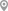Jeevan Bhima Nagar, BangaloreElectronic City, BangaloreAshapur, VaranasiMotijhil, KolkataElectronic City, BangaloreRajbari Post Office, Kolkata

X

Post your Learning Need

Let us shortlist and give the best tutors and institutes.

or

Send Enquiry to Annu

Let Annu know you are interested in their class

Reply to 's review

Enter your reply*

1500/1500

Please enter your reply

Your reply should contain a minimum of 10 charactersYour reply has been successfully submitted.

UrbanPro.com is India's largest network of most trusted tutors and institutes. Over 25 lakh students rely on UrbanPro.com, to fulfill their learning requirements across 1,000+ categories. Using UrbanPro.com, parents, and students can compare multiple Tutors and Institutes and choose the one that best suits their requirements. More than 6.5 lakh verified Tutors and Institutes are helping millions of students every day and growing their tutoring business on UrbanPro.com. Whether you are looking for a tutor to learn mathematics, a German language trainer to brush up your German language skills or an institute to upgrade your IT skills, we have got the best selection of Tutors and Training Institutes for you. Read more